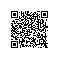# 阿里云1M带宽实际下载速度是多少？（带宽和下载速度的关系）

## 阿里云服务器1M带宽实际下载速度计算

1M带宽下载速度计算公式：1Mbit/s = 1024Kbit/s = 128KByte/s

## 带宽和下载速度对照表

1M宽带 128KB/秒
2M宽带 2*128=256KB/秒
3M宽带 3*128=384KB/秒
4M宽带 4*128=512KB/秒
5M宽带 5*128=640KB/秒
6M宽带 6*128=768KB/秒
7M宽带 7*128=896KB/秒
8M宽带 8*128=1024KB/S=1M/秒
9M宽带 9*128=1152KB/秒=1.125M/秒
10M宽带 10*128=1280KB/秒=1.25M/秒
20M宽带 20*128=2560KB/秒=2.5M/秒
50兆宽带 50*128=6400KB/秒=6.25M/秒
100兆宽带 100*128=12800KB/秒=12.5M/秒
200兆宽带 200*128=25600KB/秒=25M/秒
N兆宽带 N*128，以此类推使用钉钉扫一扫加入圈子
+ 订阅